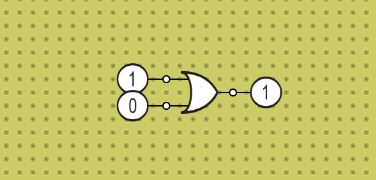# How to use logic gates

Logic gates are the Digital Electronic circuit which are used to perform logical operations and tasks.

The Logic Gates operate on Boolean functions to give the logical output for one or more than one logical inputs. The Boolean function uses the one or more than one binary input and produces a new binary output according to the function applied.

Below is the method which shows that how to use logic gates to build your logic circuit and also how to test them with their corresponding truth table.

## Steps to use a logic gate in Lab

In the below step we’re taking an or gate in order to demonstrate that how to use a logic gate in our virtual lab.

Step 1 : Scroll through the list of the components on the above and navigate to the logic gates as shown in the screen shots below.Step 2 : Click on the logic gate you want to use from the list. The logic gate will appear on the board as shown in the screenshot below.Step 3 : Now you will need to grab the input as well as the output for the logic gatelogical input for logic gateLogic output for the logic gate

Just similar to the step 2 if you click on the input and the out icon, they will appear on the board.

Step 4 : Connect the input as well as the output to your logic gates. There are the two ways you can give them a connection.

1. By directly connecting the input as well as the output to the logic gate.2. By using the wires to connect the input as well as the output to the logic gate.### Truth tables for the different logic gates

Below are the list of logic gate truth tables which might be helpful for you to keep it up while working with the logic gates.

 Truth Table for AND Gate A (Input 1) B (Input 2) A.B = Y (Output) 0 0 0 0 1 0 1 0 0 1 1 1

 Truth Table for OR Gate A (Input 1) B (Input 2) A+B = Y (Output) 0 0 0 0 1 1 1 0 1 1 1 1

 Truth Table for NOT Gate A (Input 1) A (Output) 0 1 1 0

 Truth Table for NAND Gate A (Input 1) B (Input 2) A.B = Y (Output) 0 0 0 0 1 1 1 0 1 1 1 1

 Truth Table for NOR Gate A (Input 1) B (Input 2) A+B = Y (Output) 0 0 1 0 1 0 1 0 0 1 1 0

 Truth Table for EXOR Gate A (Input 1) B (Input 2) A⊕B = Y (Output) 0 0 0 0 1 1 1 0 1 1 1 0

 Truth Table for EXNOR Gate A (Input 1) B (Input 2) A⊕B = Y (Output) 0 0 1 0 1 0 1 0 0 1 1 1

•
•
•
•
•
•